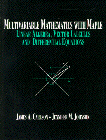Multivariable Mathematics with Maple. Linear Algebra, Vector Calculus and Differential Equations - Maplesoft Books - Maple Books, Maple Resources and Math BooksHome : Maplesoft Books : Multivariable Mathematics with Maple. Linear Algebra, Vector Calculus and Differential EquationsDetails Search# Multivariable Mathematics with Maple. Linear Algebra, Vector Calculus and Differential Equations

## 1997